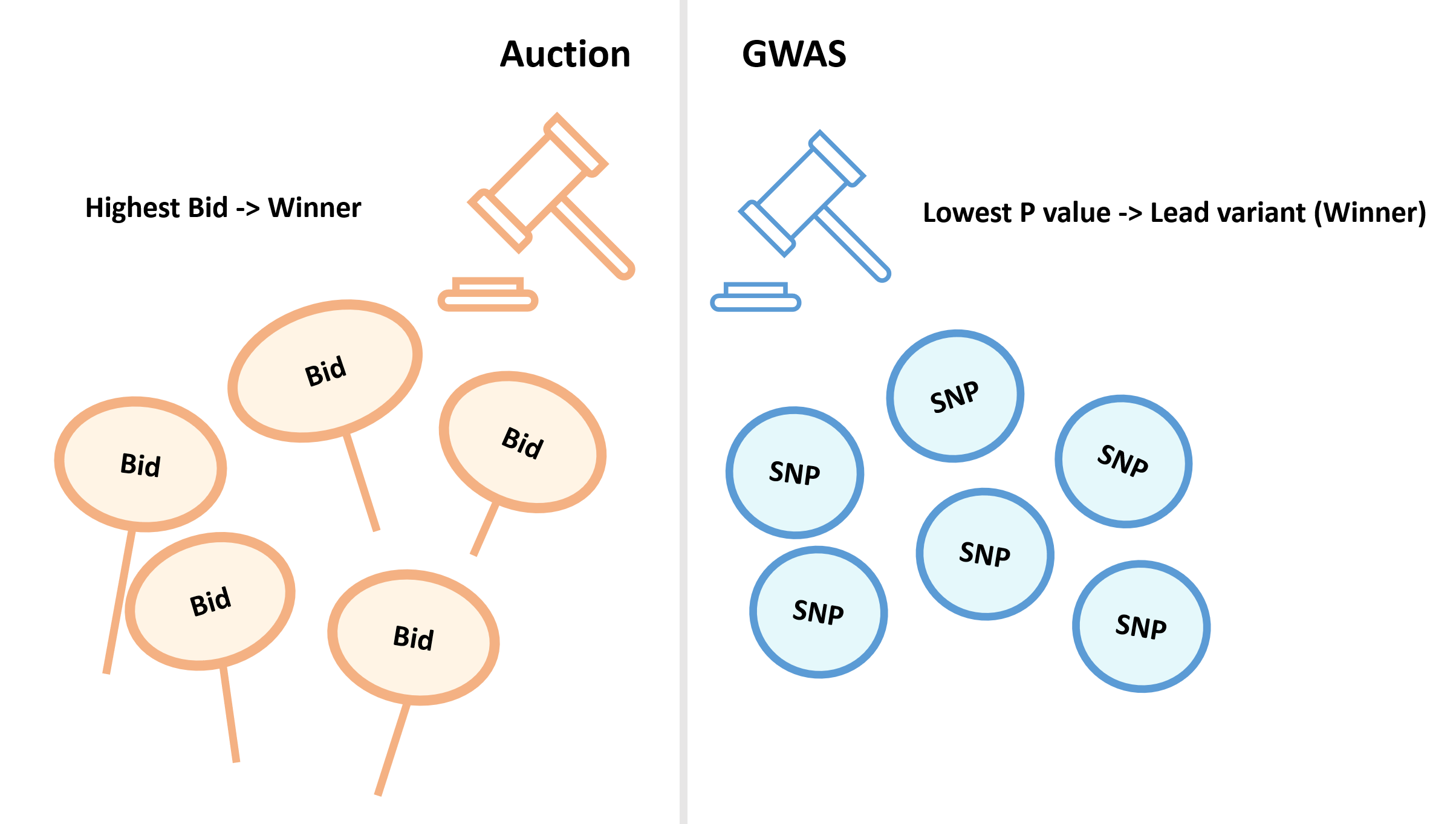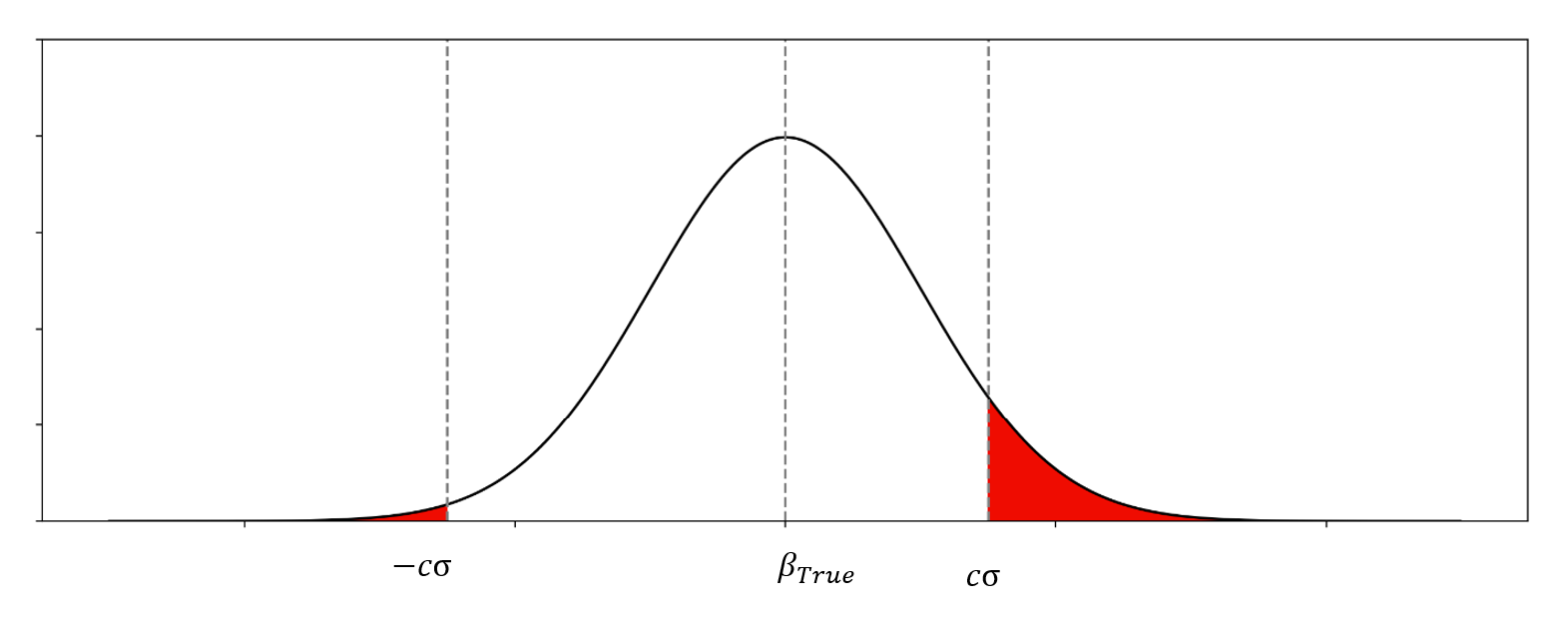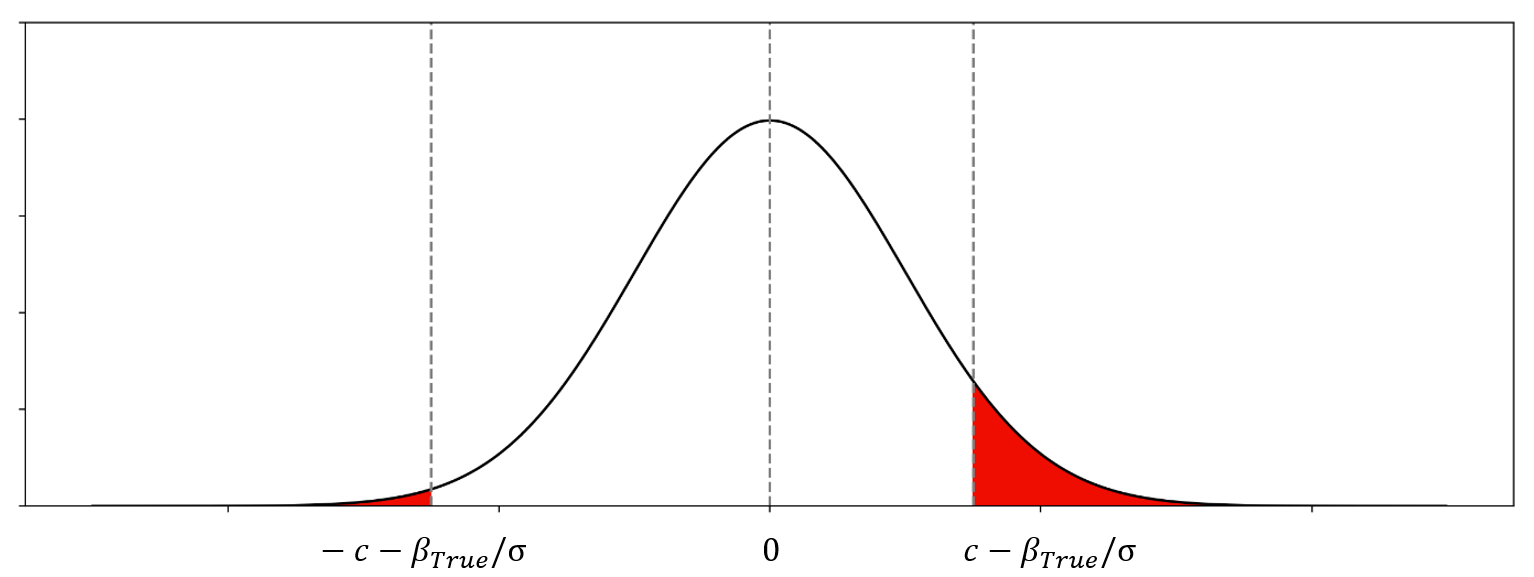## GWAS中的赢家诅咒 Winner’s curse## 赢家诅咒的校正 WC correction$\beta_{Observed} \sim N(\beta_{True},\sigma^2)$$\beta_{Observed}$的一个例子• $c$ : 显著性阈值对应的Z分数${{\beta_{Observed} - \beta_{True}}\over{\sigma}} \sim N(0,1)$${{\beta_{Observed} - \beta_{True}}\over{\sigma}}$的一个例子$f(x,\beta_{True}) ={{1}\over{\sigma}} {{\phi({{{x - \beta_{True}}\over{\sigma}}})} \over {\Phi({{{\beta_{True}}\over{\sigma}}-c}) + \Phi({{{-\beta_{True}}\over{\sigma}}-c})}}$$|{{x}\over{\sigma}}|\geq c$

•$\phi(x)$ : 标准正态分布的概率密度函数
•$\Phi(x)$ : 标准正态分布的累积分布函数$E(\beta_{Observed}; \beta_{True}) = \beta_{True} + \sigma {{\phi({{{\beta_{True}}\over{\sigma}}-c}) - \phi({{{-\beta_{True}}\over{\sigma}}-c})} \over {\Phi({{{\beta_{True}}\over{\sigma}}-c}) + \Phi({{{-\beta_{True}}\over{\sigma}}-c})}}$

•$\beta_{Observed}$ is biased.
• 偏差的大小由$\beta_{True}$, SE$\sigma$, 以及用于筛选的显著性阈值决定.

## winnerscurse R包

https://amandaforde.github.io/winnerscurse/articles/winners_curse_methods.html

## 参考

• Bazerman, M. H., & Samuelson, W. F. (1983). I won the auction but don’t want the prize. Journal of conflict resolution, 27(4), 618-634.
• Göring, H. H., Terwilliger, J. D., & Blangero, J. (2001). Large upward bias in estimation of locus-specific effects from genomewide scans. The American Journal of Human Genetics, 69(6), 1357-1369.
• Zhong, H., & Prentice, R. L. (2008). Bias-reduced estimators and confidence intervals for odds ratios in genome-wide association studies. Biostatistics, 9(4), 621-634.
• Ghosh, A., Zou, F., & Wright, F. A. (2008). Estimating odds ratios in genome scans: an approximate conditional likelihood approach. The American Journal of Human Genetics, 82(5), 1064-1074.

## GWAS方法 – SAIGE 校正样本对照失衡的广义线性混合模型

SAIGE开发背景
SAIGE原理简介
第一步
第二步
注意事项
SAIGE使用方法
安装
第一步
第二步
结果解读



① SAIGE开发背景：

SAIGE便是针对此问题开发的方法，目前UKBB等GWAS概括性数据所使用的方法均为SAIGE。SAIGE的特点是利用 SPA（saddlepoint approximation ， 鞍点近似法） 在病例对照比例严重失衡时，仍然获得准确的p值。

② SAIGE原理简介：

SAIGE全称是 Scalable and Accurate Implementation of GEneralized mixed model 广义混合模型的可扩展且准确的实现。

SAIGE 与 bolt-lmm 或 regenie 类似，都需要两个步骤。saige基于广义线性混合模型GLMM模型：

SAIGE通过PQL方法 和 AI-REML 算法简化计算提高效率。

SAIGE假设真随机效应b已经给定，并计算两种情况下T的方差的比值，来进行简化计算

saige中会取随机取30个snp来估计r。

SAIGE整体架构如下所示：

③ SAIGE的注意事项：

④ SAIGE使用方法：

saige的github页面：https://github.com/weizhouUMICH/SAIGE

saige是基于R的软件，有多种安装方法：

docker pull wzhou88/saige:0.44.2



2 表性文件

3 协变量文件

#For Binary traits:
Rscript step1_fitNULLGLMM.R     \
--phenoFile=./input/pheno_1000samples.txt_withdosages_withBothTraitTypes.txt \
--phenoCol=y_binary \
--covarColList=x1,x2 \
--sampleIDColinphenoFile=IID \
--traitType=binary        \
--outputPrefix=./output/example_binary \
--LOCO=FALSE \
--IsOverwriteVarianceRatioFile ## v0.38. Whether to overwrite the variance ratio file if the file already exists

#For Quantitative traits, if not normally distributed, inverse normalization needs to be specified to be TRUE --invNormalize=TRUE
Rscript step1_fitNULLGLMM.R     \
--phenoFile=./input/pheno_1000samples.txt_withdosages_withBothTraitTypes.txt \
--phenoCol=y_quantitative \
--covarColList=x1,x2 \
--sampleIDColinphenoFile=IID \
--traitType=quantitative       \
--invNormalize=TRUE	\
--outputPrefix=./output/example_quantitative \
--LOCO=FALSE	\\
--tauInit=1,0



• .rda文件，包含空模型
• 30个随机选择的SNP的关联结果
• 包含估计的方差比值的文本文件 （回忆上述SAIGE算法中的r

.rda文件，包含空模型

./output/example_quantitative.rda

#open R
R
#load the model file in R
names(modglmm)
modglmm\$theta

#theta: a vector of length 2. The first element is the dispersion parameter estimate and the second one is the variance component parameter estimate
#coefficients: fixed effect parameter estimates
#linear.predictors: a vector of length N (N is the sample size) containing linear predictors
#fitted.values: a vector of length N (N is the sample size) containing fitted mean values on the original scale
#Y: a vector of length N (N is the sample size) containing final working vector
#residuals: a vector of length N (N is the sample size) containing residuals on the original scale
#sampleID: a vector of length N (N is the sample size) containing sample IDs used to fit the null model



30个随机选择的SNP的关联结果

less -S ./output/example_quantitative_30markers.SAIGE.results.txt



less -S ./output/example_quantitative.varianceRatio.txt



1 插补后的剂量文件，如VCF，BGEN，BCF或SAV，以及

2 对应的索引文件

4 第一步所得的rda模型文件

5 第一步所得的方差比值文件

#bgen for dosages
Rscript step2_SPAtests.R \
--bgenFile=./input/genotype_100markers.bgen \
--bgenFileIndex=./input/genotype_100markers.bgen.bgi \
--minMAF=0.0001 \ #最小MAF不能低于0.0001
--minMAC=1 \ #最小MAC不能低于1
--sampleFile=./input/samplefileforbgen_10000samples.txt \
--GMMATmodelFile=./output/example.rda \
--varianceRatioFile=./output/example.varianceRatio.txt \
--SAIGEOutputFile=./output/example.SAIGE.bgen.txt \
--numLinesOutput=2 \
--IsOutputAFinCaseCtrl=TRUE

#VCF containing dosages (--vcfField=DS)
Rscript step2_SPAtests.R \
--vcfFile=./input/dosage_10markers.vcf.gz \
--vcfFileIndex=./input/dosage_10markers.vcf.gz.tbi \
--vcfField=DS \
--chrom=1 \
--minMAF=0.0001 \   #最小MAF不能低于0.0001
--minMAC=1 \   #最小MAC不能低于1
--sampleFile=./input/sampleIDindosage.txt \
--GMMATmodelFile=./output/example.rda \
--varianceRatioFile=./output/example.varianceRatio.txt \
--SAIGEOutputFile=./output/example.SAIGE.vcf.dosage.txt \
--numLinesOutput=2 \
--IsOutputAFinCaseCtrl=TRUE



CHR: chromosome
POS: genome position
SNPID: variant ID
Allele1: allele 1
Allele2: allele 2
AC_Allele2: allele count of allele 2
AF_Allele2: allele frequency of allele 2
imputationInfo: imputation info. If not in dosage/genotype input file, will output 1
N: sample size
BETA: effect size of allele 2
SE: standard error of BETA
Tstat: score statistic of allele 2

#SPA后的p值
p.value: p value (with SPA applied for binary traits)

p.value.NA: p value when SPA is not applied (only for binary traits)
Is.SPA.converge: whether SPA is converged or not (only for binary traits)
varT: estimated variance of score statistic with sample relatedness incorporated
varTstar: variance of score statistic without sample relatedness incorporated
AF.Cases: allele frequency of allele 2 in cases (only for binary traits and if --IsOutputAFinCaseCtrl=TRUE)
AF.Controls: allele frequency of allele 2 in controls (only for binary traits and if --IsOutputAFinCaseCtrl=TRUE)
Tstat_cond, p.value_cond, varT_cond, BETA_cond, SE_cond: summary stats for conditional analysis



Zhou, W. et al. (SAIGE)Efficiently controlling for case-control imbalance and sample relatedness in large-scale genetic association studies. Nat. Genet. 50, 1335–1341 (2018).

## 基于LMM的一种快速的关联检验方法 fastGWA

TL；DR

fastGWA的LMM模型：

fastGWA-REML 算法：grid search（加速的重点）

Jiang L, Zheng Z, Qi T, et al. A resource-efficient tool for mixed model association analysis of large-scale data[J]. Nature genetics, 2019, 51(12): 1749-1755.

https://cnsgenomics.com/software/gcta/#Overview

## 基于高斯混合模型的关联检验 Bolt-LMM – GWAS方法

Key words:　LMM，高斯混合模型，贝叶斯，无穷小模型，长尾分布

TL；DR

Bolt-LMM对SNP的效应拟合高斯混合模型，该算法使用一种快速的方差近似方法，计算近似的表型残差，并通过回顾性的分数检验统计量检验残差与待检验SNP的关联，这样就构建了表型预测的贝叶斯模型与频率学派关联检验的桥梁。同时，基于LD分数回归，还会对统计量进行调整。

• 需要大量的计算资源，时间复杂度高。
• 现有模型由于对遗传结构非最优化（suboptimal modeling assumptions regarding the genetic architectures）的假设，会导致power降低。当前标准的线性混合模型是基于无穷小模型（infinitesimal model），该模型假设所有的变异都是效应量很小的因果变异，各效应量相互独立，服从高斯分布，但实际情况中，对于复杂表型，因果loci的数量大约为1000个左右。

BOLT-LMM算法包含四个步骤，每一步都需要若干次时间复杂度为O(MN)的迭代。 (1a) 估计方差系数； (1b) 计算无穷小混合模型下的关联统计量 （Bolt-LMM-inf） (2a) 估计高斯混合模型的系数 (2b) 计算高斯混合模型下的关联统计量 （Bolt-LMM）

Y是表型，x是待检验的SNP，g是遗传效应，e是环境因素 在无穷小模型下，遗传效应g可以表示为：

σg2是方差系数。 环境效应也被认为是独立同分布，服从多元正态分布，

σe2是方差系数，I是单位矩阵。 实际上σg2与σe2是未知的，所以我们要先通过REML来估计。然后计算前瞻性的卡方检验统计量：

BOLT-LMM-inf 无穷小混合模型统计量：

cinf是一个常数的校正因子，由下式估计：

BOLT-LMM 高斯混合模型关联统计量：

Loh, P. R. et al. Efficient Bayesian mixed-model analysis increases association power in large cohorts. Nature Genetics 47, 284–290 (2015).

## Regenie – 高效的全基因组回归

• 1 相比其他方法 更快，更省内存。
• 2 可适用于数量表型，或是二分类表性。
• 3 可以同时对多个表型进行检验。
• 4 可以矫正在case-control不平衡时，mac过低造成的SNP效应值的过高估计（SAIGE-SPA存在此问题）。

regenie 方法简介：

regenie与saige或fastGWA一样，同样采用了两个步骤的模式来进行检验。

STEP 1，估计空模型 （主要利用 Stacked block ridge regression的方法）

1 层叠区块脊回归 Stacked block ridge regression

1.1 分割区块 Block partitions

1.2 0级脊回归 Level 0 ridge regressions

1.3 1级脊回归 Level 1 ridge regression

1.3 1级脊回归 Level 1 ridge regression

1.5 LOCO

1.6 对于二分类表型，

STEP 2 关联检验 Association testing

2.1 对于数量表型，考虑一下模型，

2.2 对于二分类表型，采用以下模型

2.3 Firth logistic regression（重点）

2.4 regnie在第二步中也支持saige所使用的SPA方法。这里就略过。

https://rgcgithub.github.io/regenie/

https://www.biorxiv.org/content/10.1101/2020.06.19.162354v2